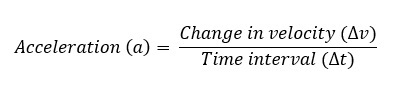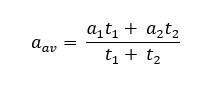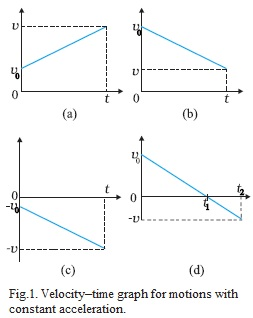The time rate of change of velocity is called acceleration.Its unit is m/s2. Its dimensional formula is [M0 L T-2]. It is a vector quantity.

Acceleration can be positive, zero or negative.

• Positive acceleration means velocity increasing with time.
• Zero acceleration means velocity is uniform.
• Negative acceleration (retardation)means velocity is decreasing with time.If a particle is accelerated for a time t1 with acceleration a1 and for a time t2 with acceleration a2, then average accelerationVelocity–time graph for motions with constant acceleration:In Fig.1 Graph (a) Motion in positive direction with positive acceleration,

Graph (b) Motion in positive direction with negative acceleration,

Graph (c) Motion in negative direction with negative acceleration,

Graph (d) Motion of an object with negative acceleration that changes direction at time t1. Between times 0

to t1, its moves in positive x – direction and between t1 and t2 it moves in the opposite direction.